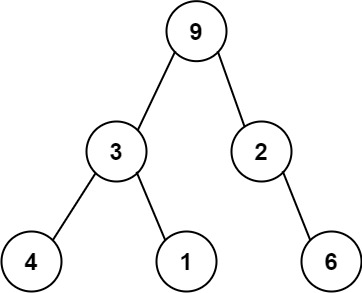304 North Cardinal St.
Dorchester Center, MA 02124

# Verify Preorder Serialization of a Binary Tree LeetCode Solution

## Problem – Verify Preorder Serialization of a Binary Tree LeetCode Solution

One way to serialize a binary tree is to use preorder traversal. When we encounter a non-null node, we record the node’s value. If it is a null node, we record using a sentinel value such as `'#'`.For example, the above binary tree can be serialized to the string `"9,3,4,#,#,1,#,#,2,#,6,#,#"`, where `'#'` represents a null node.

Given a string of comma-separated values `preorder`, return `true` if it is a correct preorder traversal serialization of a binary tree.

It is guaranteed that each comma-separated value in the string must be either an integer or a character `'#'` representing null pointer.

You may assume that the input format is always valid.

• For example, it could never contain two consecutive commas, such as `"1,,3"`.

Note: You are not allowed to reconstruct the tree.

Example 1:

``````Input: preorder = "9,3,4,#,#,1,#,#,2,#,6,#,#"
Output: true
``````

Example 2:

``````Input: preorder = "1,#"
Output: false
``````

Example 3:

``````Input: preorder = "9,#,#,1"
Output: false
``````

Constraints:

• `1 <= preorder.length <= 104`
• `preorder` consist of integers in the range `[0, 100]` and `'#'` separated by commas `','`.

## Verify Preorder Serialization of a Binary Tree LeetCode Solution in Java

``````public boolean isValidSerialization(String preorder) {
String[] nodes = preorder.split(",");
int diff = 1;
for (String node: nodes) {
if (--diff < 0) return false;
if (!node.equals("#")) diff += 2;
}
return diff == 0;
}
``````

## Verify Preorder Serialization of a Binary Tree LeetCode Solution in Python

``````class Solution(object):
def isValidSerialization(self, preorder):
"""
:type preorder: str
:rtype: bool
"""
# remember how many empty slots we have
# non-null nodes occupy one slot but create two new slots
# null nodes occupy one slot

p = preorder.split(',')

#initially we have one empty slot to put the root in it
slot = 1
for node in p:

# no empty slot to put the current node
if slot == 0:
return False

# a null node?
if node == '#':
# ocuppy slot
slot -= 1
else:
# create new slot
slot += 1

#we don't allow empty slots at the end
return slot==0
``````

## Verify Preorder Serialization of a Binary Tree LeetCode Solution in C++

``````class Solution {
public:
bool isValidSerialization(string preorder) {
if (preorder.empty()) return false;
preorder+=',';
int sz=preorder.size(),idx=0;
int capacity=1;
for (idx=0;idx<sz;idx++){
if (preorder[idx]!=',') continue;
capacity--;
if (capacity<0) return false;
if (preorder[idx-1]!='#') capacity+=2;
}
return capacity==0;
}
};
``````
##### Verify Preorder Serialization of a Binary Tree LeetCode Solution Review:

In our experience, we suggest you solve this Verify Preorder Serialization of a Binary Tree LeetCode Solution and gain some new skills from Professionals completely free and we assure you will be worth it.

If you are stuck anywhere between any coding problem, just visit Queslers to get the Verify Preorder Serialization of a Binary Tree LeetCode Solution

Find on Leetcode

##### Conclusion:

I hope this Verify Preorder Serialization of a Binary Tree LeetCode Solution would be useful for you to learn something new from this problem. If it helped you then don’t forget to bookmark our site for more Coding Solutions.

This Problem is intended for audiences of all experiences who are interested in learning about Data Science in a business context; there are no prerequisites.

Keep Learning!

More Coding Solutions >>

LeetCode Solutions

Hacker Rank Solutions

CodeChef Solutions